# Coarse Harmonic Motion

Is it possible that “Matter” is simply “Incompressible Energy”

Coarse Harmonic Motion (CHM) would be the type of motion undertaken by a Coarse Harmonic Oscillator, an oscillator similar to a Simple Harmonic Oscillator but instead of pure continuous motion – this oscillator’s motion back and forth occurs in Coarse Incremental Steps…

Coarse Harmonic Motion (CHM) would be the type of motion undertaken by a Coarse Harmonic Oscillator, an oscillator similar to a Simple Harmonic Oscillator but uses discrete step-sizes instead of pure continuous motion – a Coarse Step-Size of Oscillation.

We will here examine what happens to the behaviour of a harmonic oscillator as we increase the “coarseness” of the increment step-size of oscillation.

ω1 is One Degree

For ease of discussion, let’s say the fundamental unit of time is 1 second and the fundamental angular step-size (ω) is one degree per unit time – thus 1 degree per second translates to a fundamental angular frequency of 0.01745 radians per unit time.

ħ =1/57      Ħ =57      di = (1 + i 1/57)

and    di(1Ħ)   =   (1 + i 1/57)   =   1 Radian

and    di(2πĦ)   =   (1 + i 1/57)2πĦ   =   6.28 Radians

Since 1 degree is approximately 1/57 of one Radian (the unit of angular frequency) and exactly 1/360 of one Cycle/Oscillation (the unit of frequency); then this means that the fundamental frequency is 0.00278 oscillations per unit time, and the fundamental wavelength (in time) is 360 steps of 1 degree (or 360 steps of 0.01745 radians which is equal to 2π Radians)!

[Note:   f  =  ω/2π   =   0.01745/2π   =   0.00278 oscillations per unit time]  [Note:  360 steps of step-size 0.01745 radians is equal to a total of 2π Radians]

Here is the graph of that evolution as the system evolves at a step-size of one degree per unit time.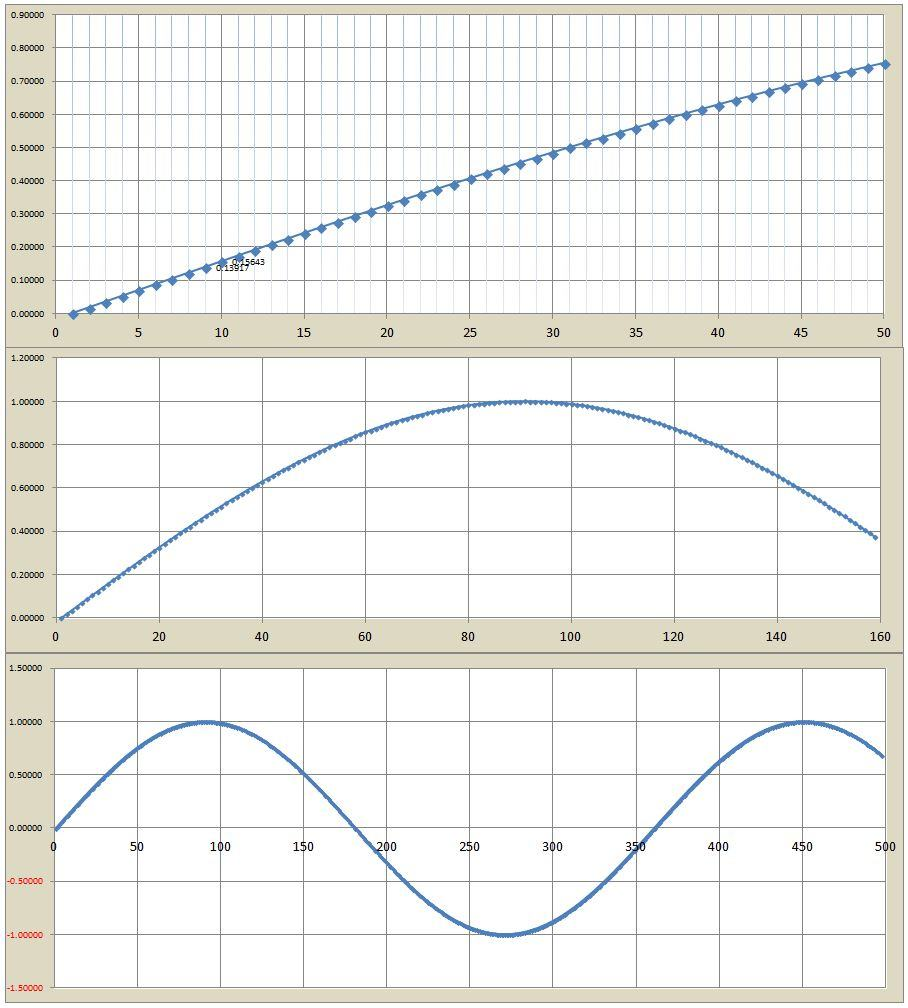Now we double the step size (step-size = 2 Degrees)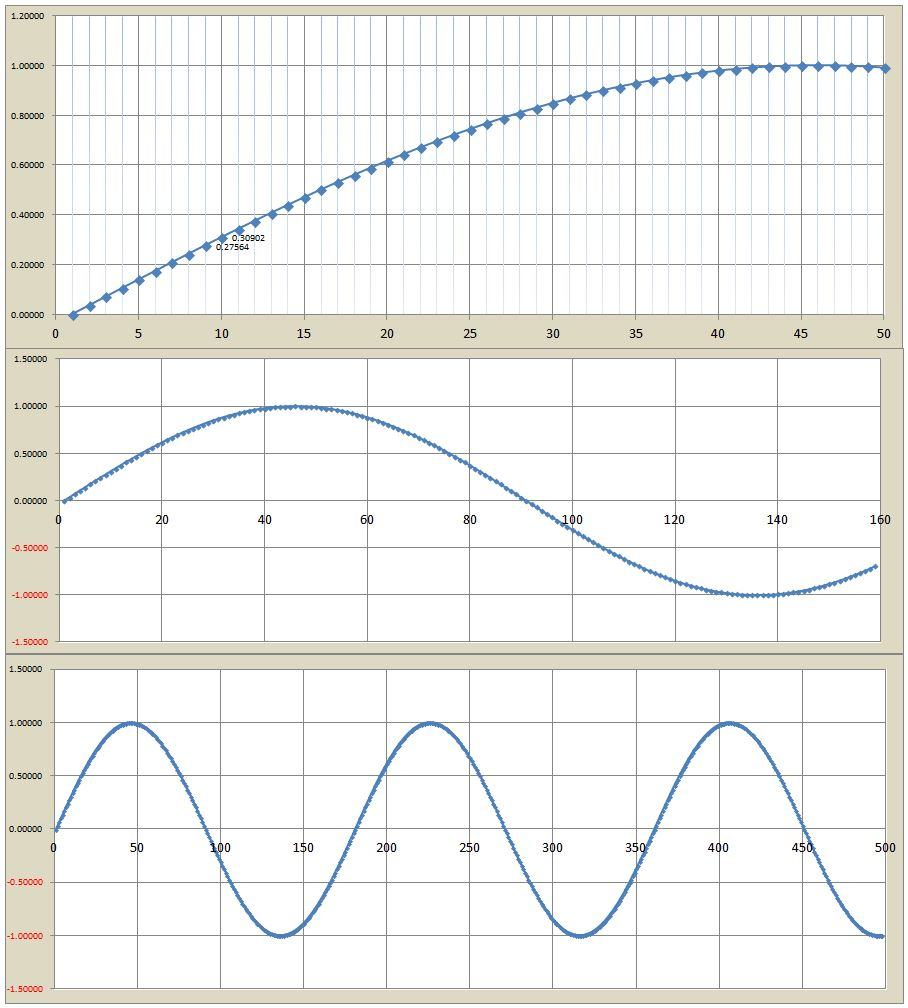Now let’s make it 10 times the fundamental step size (step-size = 10 Degrees)Now let’s make it 33 times the fundamental step size (step-size = 33 Degrees)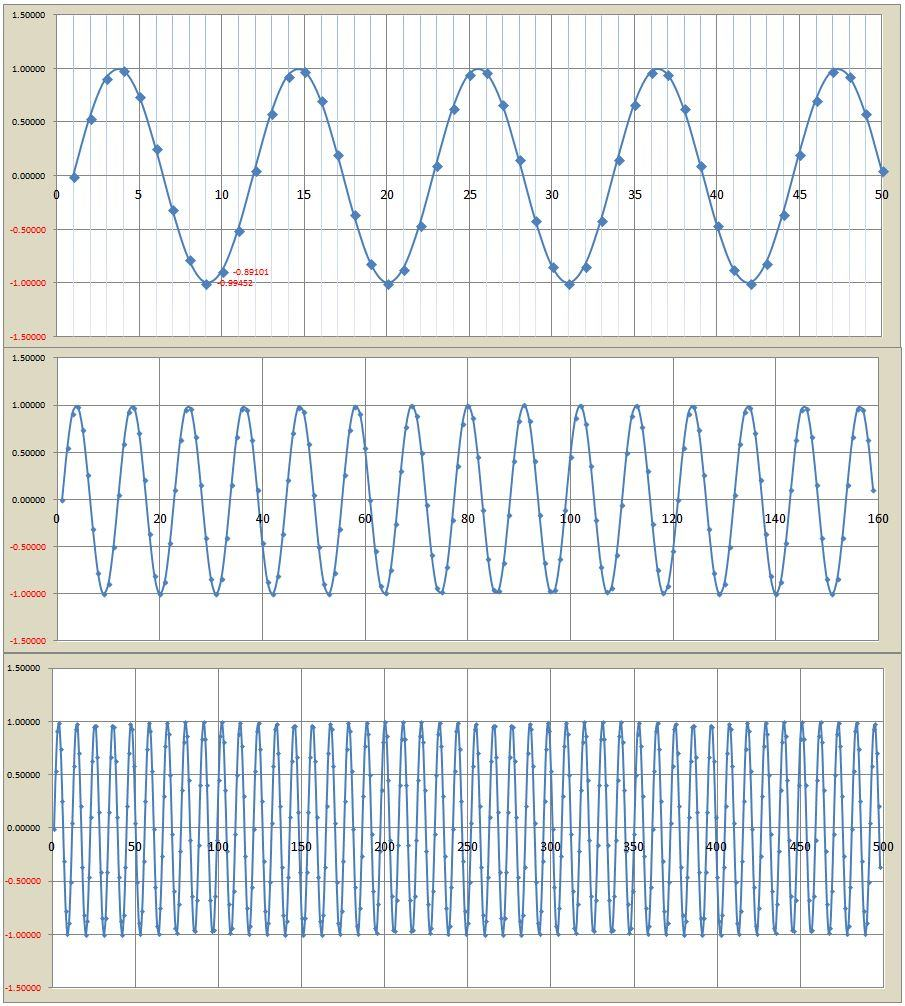Now let’s make it 73 times the fundamental step size (step-size = 73 Degrees)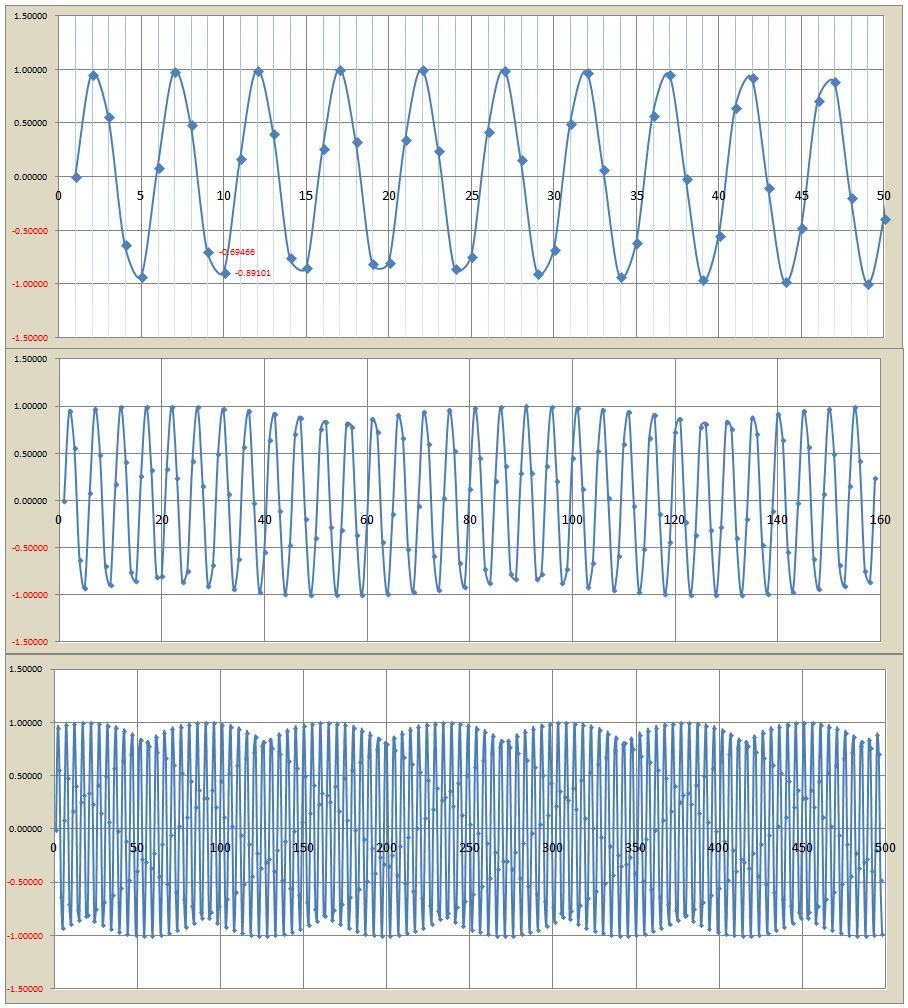Now let’s make it 134 times the fundamental step size (step-size = 134 Degrees)Now let’s make it 154 times the fundamental step size (step-size = 154 Degrees)Now let’s make it 166 times the fundamental step size (step-size = 166 Degrees)Now let’s make it 172 times the fundamental step size (step-size = 172 Degrees)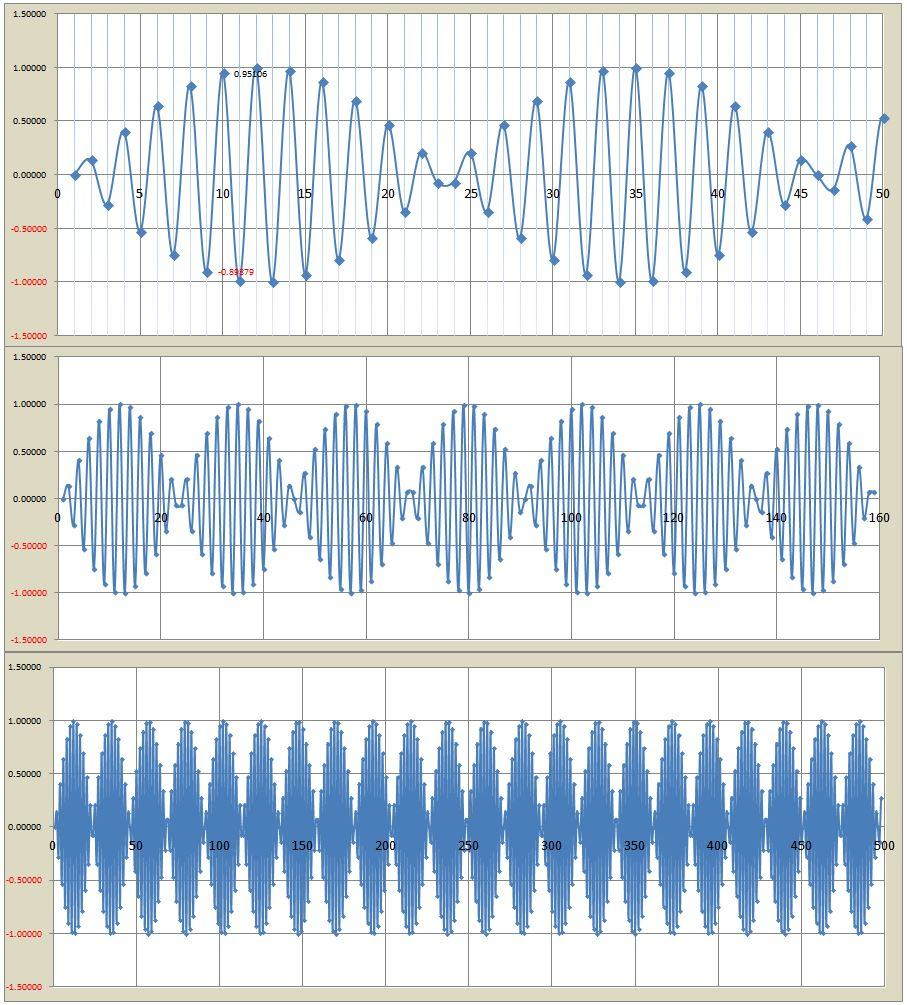Finally let’s make it 179 times the fundamental step size (step-size = 179 Degrees)Analysis of Waveforms

As we can see, for small step-size, everything appears as expected, smooth harmonic motion.  However as we increased the step-size, the synchronicity of oscillation (i.e. the ability of the oscillation to synchronize the starting point of a new cycle with the starting point of the previous cycle) began to be disrupted and as a result imbalances begin to emerge.  Further increases in phase-size/step-size cause some curious patterns to emerge, and finally at high degree of coarseness, very well defined “beats” eventually emerge.

So why is this interesting?  It is interesting because it shows that high energy “coarse oscillations” exhibit “matter-like properties”.  Coarse Harmonic Motion seems to suggest that symmetry-breaking, and its resultant complexity in structure, can emerge from coarse synchronization in time.